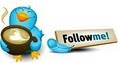#Summarize an existing summarize table• ### General discussion

•hi All,

I'm new in DAX, and face a problem about SUMMARIZE function.

====================

First I use summarize an table 'A' group by cust_id and max(value) --> SUMMARIZE('A', [cust_id],"max_value",max(value))

 cust_id value A 2 A 3 B 1 C 1 C 3

====================

And the result will be OK as below,

 cust_id value A 3 B 1 C 3

====================

And then, I need to summarize this table group by value and count(cust_id) but don't know how to do....it cannot recognize existing 'cust_id' column...

the result I expect is,

 value count 1 1 3 2

Jackie

Monday, November 12, 2012 8:52 AM

### All replies

•Instead of using summarize, why don't you use 2 measures:

Max_Value: =Max([value])

Count: =countx('A';[Max_Value])

The result will be something like:

 Max_Value Count A 3 2 B 1 1 C 3 2

You can then create another pivotable based on the first one by using OLAP ... convert into formula:

 Max_Value Min of Count 1 1 3 2

Monday, November 12, 2012 11:22 AM
•I am not really clear why you need two summarize for this? Do you need this in PowerPivot or are you creating it a DAX query? If it is in PowerPivot, I would say to create a calculated column for the value and then a measure for the count. The reason value needs to be a calculated column is because you need to drag and drop it in the rows. Follow the steps below:-

1) For the purpose of this demo, I have imported the rows that you gave as a linked table and is called Table1. Create a calculated column called MaxValue with the formula below:-

`=calculate(max(Table1[value]), filter(Table1, EARLIER(Table1[cust_id])=Table1[cust_id]))`

2) Now save it and then create a measure called CntMaxValue with the formula below

`=distinctcount(Table1[cust_id])`

3) Preview the results by putting MaxValue in rows and CntMaxValue in valuesCheers,
Jason | www.SqlJason.comMonday, November 12, 2012 2:04 PM
•Hi Jason & NGUYEN,

Thanks for your reply, and let me clarify my request for you.

here is the fact table,

 cust_id value A 2 A 3 B 1 C 1 C 3

And the dimension table(Dim),

 D_value 1 2 3

And I need to create a measurement(cust count) when drag it into pivot table, it'll look like,

 D_value cust count 1 1 3 1

This measurement need to find the max(value) by customer(1st summarize) and then count by each value(2nd summarize).

I can do 1st summarize like below, but have no idea how to do 2nd summarize in the outside...

SUMMARIZE(
CALCULATETABLE
('A', ALL('Dim'[D_value]))
,[cust_id]
,"max_value"
, max('A'[value])
)

please feel free to let me know if any questions.

Jackie

• Edited by Tuesday, November 13, 2012 2:16 AM
Tuesday, November 13, 2012 2:14 AM
•do you need it as a result of a dax query? Where is this being used?

Because the method I showed will give you the results when you use the measure...

Are you using the tabular model? or PowerPivot?

Cheers,
Jason | www.SqlJason.comTuesday, November 13, 2012 2:31 AM
•hi Jason,

I use tabular model; I need to count the summarize table, so I will add countrows outside of summarize result.

I tried your sample to create a static column and use distinctcount, but I think my case will be dynamic depend on what user drag into.

Because the fact table also include other columns(like Product, Country...), so if pre-create this column it will not be correct.

Jackie

Tuesday, November 13, 2012 3:27 AM
•Jackie,

Is this still an issue?

Thanks!

Ed Price, Power BI & SQL Server Customer Program Manager (Blog, Small Basic, Wiki Ninjas, Wiki)